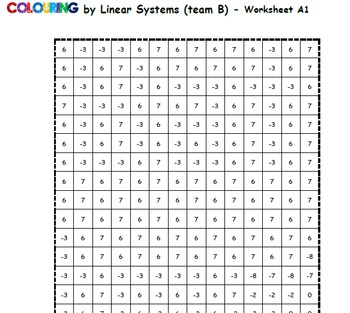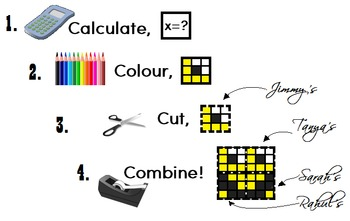# Batman v Superman Math Mosaic Battle - Product MenuSubject
Resource Type
File Type
PDF (1 MB|16 pages)
Standards
FREE
• Product Description
• Standards
Engage your whole class in a highly creative and highly mathy heroic colouring battle! I would like to introduce my latest line of collaborative math mosaics, and allow you to see the various versions available, all in one easy-to-navigate file.

Basically, students each have a small colour-by-number grid that represents a section of the whole-class mosaic. Quite a bit of math must be done to determine which colour each number should be! The worksheets combine to create the large superhero mosaics.

It's simple!
2. Colour the squares.
4. Combine with the class!

Have a look at the five versions presented in this PDF (.pdf) document, and navigate within the document easily using the hyperlinks to see sample worksheets.

In the full products, full class sets of 12 worksheets are provided for both the Batman and Superman mosaics, so you could split up your students and have a colouring showdown of epically mathematical proportions! May the best coloured hero win!

Each mosaic consists of 12 worksheets, each with 12 problems whose solutions decode the colour-key. The collaborative motivation is huge!

The answers are given in a randomized list at the bottom of each sheet, allowing students to monitor their own work. Complete answer keys are provided for you, as well as a Teaching Tips page for smooth implementation!

Although this product menu comes as a PDF file, all the full products include both .docx and .pdf versions.

The Superman vs Batman battle is available in the following versions (all displayed in this product sample):
Fractions - +,-, ×, and ÷ positive mixed fractions
Sine and Cosine Laws - solve for sides and angles
Trig Ratios (SohCahToa) - solve for sides and angles
Pythagorean Theorem - word problems and life applications
Linear Systems - substitution, elimination, and graphing

Both a visual answer key, showing the completed mosaic with labelled coordinates (to help you assemble the picture from the combined worksheets), and a numeric answer key for each individual worksheet are provided for both hero mosaics.

Leave the hero pictures a secret, or let them know their team in advance for motivation… it’s your call.

Encourage students to check their answers by finding them in the randomized list on their worksheet before they colour each square. This will increase the accuracy of the final picture!

All my “Colouring by…” worksheets use standard pencil-crayon colours found in the Crayola 24 pack. For best results, use the exact colour name match (and encourage quality colouring!). Perhaps a class set of pencil crayons would be a fun math department investment!

Thanks for checking this out!
~CalfordMath

If you like this product, be sure the check out the whole Collaborative Math Mosaic directory, sorted by topic!
Explain why the 𝘹-coordinates of the points where the graphs of the equations 𝘺 = 𝘧(𝘹) and 𝘺 = 𝑔(𝘹) intersect are the solutions of the equation 𝘧(𝘹) = 𝑔(𝘹); find the solutions approximately, e.g., using technology to graph the functions, make tables of values, or find successive approximations. Include cases where 𝘧(𝘹) and/or 𝑔(𝘹) are linear, polynomial, rational, absolute value, exponential, and logarithmic functions.
Represent a system of linear equations as a single matrix equation in a vector variable.
Solve systems of two linear equations in two variables algebraically, and estimate solutions by graphing the equations. Solve simple cases by inspection. For example, 3𝘹 + 2𝘺 = 5 and 3𝘹 + 2𝘺 = 6 have no solution because 3𝘹 + 2𝘺 cannot simultaneously be 5 and 6.
Understand that solutions to a system of two linear equations in two variables correspond to points of intersection of their graphs, because points of intersection satisfy both equations simultaneously.
Analyze and solve pairs of simultaneous linear equations.
Total Pages
16 pages